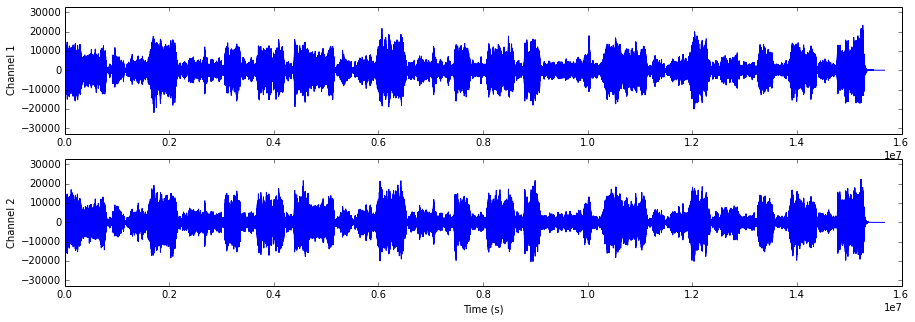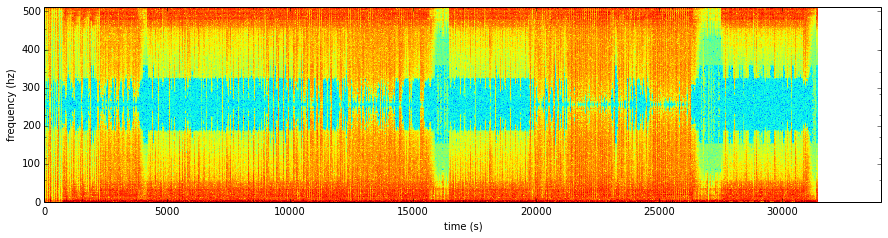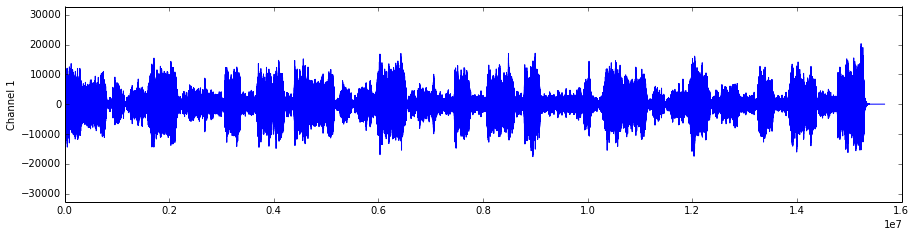# Music Information Retrieval:Part 2

## Feature Extraction

### Alexander Schindler

Research Assistant
Institute of Software Technology and Interactive Systems
Vienna University of Technology
http://www.ifs.tuwien.ac.at/~schindler

This article is a first attempt towards an interactive textbook for the Music Information Retrieval (MIR) part of the Information Retrieval lecture held at the Vienna University of Technology. The content either serves as description of basic music feature extraction as presented in the lecture as well as executable code examples that can be used and extended for the exercises.

# Introduction

A typical CD quality mainstream radio track has an average length of three minutes. This means, that the song is digitally described in Pulse-code Modulation (PCM) by allmost 16 million numbers (3 [minutes] x 60 [seconds] x 2 [stereo channels] x 44100 [sampling rate]). This information requires 30MB of memory and a considerable amount of time to process. Processing the small number of 100 tracks, which relates to about 10 audio CDs, would require about 3GB of memory, which is currently about the average size of memory provided in personal computers. Processing 100000 songs would require 3TB of memory, which requires vast ressources (e.g. aquisition, hosting, energy consumption, etc.) and is only suitable for academic or industrial settings.

Consequently, there is a strong desire to reduce the information provided in an audio track an destill it into a smaller set of representative numbers that capture higher level information about the underlying track.

# Prerequisites¶

This article is an IPython Notebook. IPython is a powerful interactive Python shell providing extensive support for data visualization and explorative experimentation. It further provides an interactive browser-based interface with support for code execution, visualization, mathematical expressions and text. This means, that if you have a running IPython Notebook server, you can download and execute this article.

## Required Environment¶

### Python¶

This article demonstrates music feature extraction using the programming language Python, which is a powerful and easy to lean scripting language, providing a rich set of scientific libraries. The examples provided have been coded and tested with Python version 2.7. Since the Python syntax varies considerably between major versions, it is recommended to use the same version.

### Python Libraries¶

The following Python libraries may be not contained in standard Python distributions and may need to be installed additionally:

• Numpy: the fundamental package for scientific computing with Python. It implements a wide range of fast and powerful algebraic functions.
• sklearn: Scikit-Learn is a powerful machine learning package for Python built on numpy and Scientific Python (Scipy).
• Scipy
• scikits.talkbox: Talkbox, a set of python modules for speech/signal processing

If you have installed all required libraries, the follwing imports should run without errors.

In :
%pylab inline

import warnings
warnings.filterwarnings('ignore')

# numerical processing and scientific libraries
import numpy as np
import scipy

# signal processing
from scipy.io                     import wavfile
from scipy                        import stats, signal
from scipy.fftpack                import fft

from scipy.signal                 import lfilter, hamming
from scipy.fftpack.realtransforms import dct
from scikits.talkbox              import segment_axis
from scikits.talkbox.features     import mfcc

# general purpose
import collections

# plotting
import matplotlib.pyplot as plt
from numpy.lib                    import stride_tricks

from IPython.display              import HTML
from base64                       import b64encode

# Classification and evaluation
from sklearn.preprocessing import StandardScaler
from sklearn import svm
from sklearn.cross_validation import StratifiedKFold, ShuffleSplit, cross_val_score
from sklearn.naive_bayes import GaussianNB
from sklearn.neighbors import KNeighborsClassifier
from sklearn.ensemble import RandomForestClassifier
from sklearn.metrics import classification_report, confusion_matrix

import pandas as pd

Populating the interactive namespace from numpy and matplotlib

WARNING: pylab import has clobbered these variables: ['hamming', 'fft']
%matplotlib prevents importing * from pylab and numpy


Some code segments need to be adapted to your local settings (e.g. the paths of the audio files) or you may test the code on different sound files. Code blocks that need to be changed are annotated with the following comment:

### change ###

## Music Files¶

A set of music files will be used to demonstrate different aspects of music feature etraction. The audio tracks used in this article were downloaded from the FreeMusicArchive and are redistributable licensed under the Creative Commons license. To visualize the expressivenmess of music features and their ability to discriminate different types of music, the songs of this article originate from different music genres.

In the code-block below the sound files used in this tutorial will be specified. Please change the paths of the files according your local settings. Because MP3-decoding is not constistently implemented across all platforms, it is required that to manually convert the audio files into wave format as a prerequiste.

In :
# initialize music collection
sound_files = collections.defaultdict(dict)

### change ###
sound_files["Classic"]["online_id"]    = 70444

sound_files["Jazz"]["path"]            = r"D:\Dropbox\Work\IFS\Lehre\Information Retrieval LVA\IPython\Michael_Winkle_-_03_-_I_Guess_I_Knew.wav"
sound_files["Jazz"]["online_id"]       = 22974

sound_files["Rock"]["path"]            = r"D:\Dropbox\Work\IFS\Lehre\Information Retrieval LVA\IPython\Room_For_A_Ghost_-_02_-_Burn.wav"
sound_files["Rock"]["online_id"]       = 61491

sound_files["Electronic"]["path"]      = r"D:\Dropbox\Work\IFS\Lehre\Information Retrieval LVA\IPython\Broke_For_Free_-_02_-_Calm_The_Fuck_Down.wav"
sound_files["Electronic"]["online_id"] = 37909

sound_files["Metal"]["path"]           = r"D:\Dropbox\Work\IFS\Lehre\Information Retrieval LVA\IPython\Acrassicauda_-_02_-_Garden_Of_Stones.wav"
sound_files["Metal"]["online_id"]      = 30919

sound_files["Rap"]["path"]             = r"D:\Dropbox\Work\IFS\Lehre\Information Retrieval LVA\IPython\Social_Studies_-_The_Wapner.wav"
sound_files["Rap"]["online_id"]        = 70602


The following code embeds the audio player from the FMA Web page into this notebook. Thus, it is possible to pre-listen the audio samples online.

In :
list_audio_samples(sound_files)

Out:
Classic

Jazz

Metal

Rap

Rock

Electronic

# Audio Representations¶

Basic knowledge of the production process of digital audio is essential to understand how to extract music features and what they express.

## Sampling and Quatization¶Audio signals as perceived by our ears have a continuous form. Analog storage media were able to preserve this continuous nature of sound (e.g. vinyl records, music cassttes, etc.). Digital logic circuits on the other hand rely on electronic oscillators that sequentially trigger the unit to process a specific task on a discrete set of data units (e.g. loading data, multiplying registers, etc.). Thus, an audio signal has to be fed in small pieces to the processing unit. The process of reducing a continuous signal to a discrete signal is called sampling. The audio signal is converted into a sequences of discrete numbers that are evenly spaced in time.

As an example one could monitor the temperature in an office by measuring every minute the current degree of Celsius. We fruther simplify this example by accepting only integer values. In this case the continous change of temparature in the office is sampled at a rate of 60 samples per minute. Since Celsius values in offices seldom rise above 128 or drop below -128 degree, it is sufficient to use 8 Bits to store the sampled data. The process of turing continous values (e.g. temperature, sound pressure, etc.) into discrete values is called quantization.

For digitizing audio especially music in CD quality, typically a sampling rate of 44100 Herz at a bit depth of 16 is used. This means, that each second of audio data is represented by 44100 16bit values.

• The time domain
• The frequency domain
• The Fourier Transform
• The Short-Time Fourier Transform

To start the feature extraction process, the audio files have to be opened and loaded. Usually audio files are opened as streams and processed sequentially, but for this tutorial it is more convenient to fully keep them in memory. After the audio data has been loaded two essential data blocks are known: the actual audio data and the rate the source has been sampled with. From this information it is easy to derive the first audio feature: the length of the track. Since the samplerate is defined as number of samples per second, the length is simply calculated by dividing the sample cound by the samplerate.

In :
# load sound files
for genre in sound_files.keys():

sound_files[genre]["samplerate"]        = samplerate
sound_files[genre]["wavedata"]          = wavedata
sound_files[genre]["number_of_samples"] = wavedata.shape
sound_files[genre]["song_length"]       = int( sound_files[genre]["number_of_samples"] / samplerate ) # round up


## The Time Domain¶

Time domain analysis is analyzing the data over a time period. Functions such as electronic signals, market behaviors, and biological systems are some of the functions that are analyzed using time domain analysis. For an electronic signal, the time domain analysis is mainly based on the voltage – time plot or the current – time plot. In a time domain analysis, the variable is always measured against time. There are several devices used to analyze data on a time domain basis. The cathode ray oscilloscope (CRO) is the most common device when analyzing electrical signals on a time domain.

### Waveform¶

A waveform is the shape and form of a signal such as a wave moving in a physical medium or an abstract representation.

In many cases the medium in which the wave is being propagated does not permit a direct visual image of the form. In these cases, the term 'waveform' refers to the shape of a graph of the varying quantity against time or distance. An instrument called an oscilloscope can be used to pictorially represent a wave as a repeating image on a screen. By extension, the term 'waveform' also describes the shape of the graph of any varying quantity against time

In :
show_stereo_waveform(sound_files["Classic"]["wavedata"]);<matplotlib.figure.Figure at 0x14eee198>

## The Frequency Domain¶

### Fourier Transform¶

• Essential part of any audio feature extraction algorithm

• Audio waves contain a spectrum of many different frequencies, each with its own amplitude and phase.

• Idea is that every complex continuous signal can be explained by decompose a wave into its component frequencies and phases.

• Inverse Fourier Transform Transform back from frequency into time domain
• No loss of data when transformation is applied

• Results of the Fourier Transform are
• Phase histogram (rarely used)
• Frequency histogram
• Sets of bins
• Each corresponding to a different range of frequencies

### Magnitude Spectrum vs. Power Spectrum¶

• the power spectrum is the magnitude spectrum squared (calculated for each bin, by summing the square of the imaginary output of the FFT with the square of the real value)
• magnitude spectrum and power spectrum rarely used directly as features (too much raw information)
• many spectral features are derived from either the power spectrum or the magnitude spectrum

### Fast Fourier Transform¶

• efficient algorithm to compute the discrete Fourier transform (DFT)
• divide and conquer algorithm
• $O(N log N)$ instead of $O(N2)$
• $N$ must be a power of 2
In :
genre = "Rock"

plotstft(sound_files[genre]["wavedata"], sound_files[genre]["samplerate"]);# Audio Pre-processing¶

Combine separate channels

In :
for genre in sound_files.keys():

if sound_files[genre]["wavedata"].shape > 1:

# use combine the channels by calculating their geometric mean
sound_files[genre]["wavedata"] = np.mean(sound_files[genre]["wavedata"] , axis=1)



Below an example waveform of a mono channel after combining the stereo channels by arithmetic mean:

In :
show_mono_waveform(sound_files["Classic"]["wavedata"])<matplotlib.figure.Figure at 0x14f0c7f0>

# Audio Features¶

## Time Domain Features¶

### Zero Crossing Rate¶

Zero-crossing rate is a simple, straightforward and inexpensive feature to examine the similarity between two or more accelerometer sensors. It measures whether two sets of time series measurements exhibit similar patters. It is particularly useful to analyze measurements that are corrupted by noise. For example, a measurement with a high zero-crossing rate, i.e., the number of samples per second that cross the zero reference line, indicates that it is noisy. However, we observe that even for sensors having the same orientation, the calibration error results in a deviation of 40s. Substituting the zero-crossing value by a mean valuecrossing, i.e., by defining a mean threshold that serves as a reference, results in a calibration error with a deviation between 2 and 15/s for individual axes and between 0 and 2/s for the absolute acceleration values of the individual measurements. Cross test of uncorrelated measurements, on the other hand, reveals that the overall calibration error is markedly bigger: between 7 and 50/s for the raw measurements and between 5 and 30/s for the absolute values.

The Zero Crossing Rate (ZCR) is defined by:

$zcr = \frac{1}{N-1} \sum_{i=1}^{N-1} | sign|x(i)| - sign|x(i-1)| |$

where $N$ is the number of samples of an audio file and the signum function is defined by:

$sign|x(i)| = \begin{cases} 1, & \text{ if } x(i) > 0\\ 0, & \text{ if } x(i) = 0\\ -1,&\text{ if } x(i) < 0 \end{cases}$

average number of times the audio signal crosses the zero amplitude line per time unit. very simple to compute has been applied to speech processing to distinguish voiced sections from noise

also has been applied to MIR tasks such as classifying percussion sounds Genres

In :
def zero_crossing_rate_BruteForce(wavedata):

zero_crossings = 0

for i in range(1, number_of_samples):

if ( wavedata[i - 1] <  0 and wavedata[i] >  0 ) or \
( wavedata[i - 1] >  0 and wavedata[i] <  0 ) or \
( wavedata[i - 1] != 0 and wavedata[i] == 0):

zero_crossings += 1

zero_crossing_rate = zero_crossings / float(number_of_samples - 1)

return zero_crossing_rate


The following method is numerical more elegant and computationally efficient. It uses the optimized linear algebraic functions of the Numerical Python (numpy) package.

The method further computes the Zero Crossing Rate for a sequence of blocks (also called frames or windows).

In :
def zero_crossing_rate(wavedata, block_length, sample_rate):

# how many blocks have to be processed?
num_blocks = int(np.ceil(len(wavedata)/block_length))

# when do these blocks begin (time in seconds)?
timestamps = (np.arange(0,num_blocks - 1) * (block_length / float(samplerate)))

zcr = []

for i in range(0,num_blocks-1):

start = i * block_length
stop  = np.min([(start + block_length - 1), len(wavedata)])

zc = 0.5 * np.mean(np.abs(np.diff(np.sign(wavedata[start:stop]))))
zcr.append(zc)

return np.asarray(zcr), np.asarray(timestamps)


The following charts visualize how the Zero Crossing Rate correlates with the audio signal. The upper chart shows the spectrogram of the track. The lower chart superimposes the Zero Crossing Rate over the waveform of the track. It can be observed that sewuences with increased energy in high frequency reagions have a higher zero crossing rate.

In :
genre = "Rock"

# calculate zero-crossing-rate
zcr, ts = zero_crossing_rate(sound_files[genre]["wavedata"], 2048, sound_files[genre]["samplerate"]);

show_feature_superimposed(genre, zcr, ts);